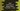# Java Program to print the sum of square series 1^2 +2^2 + ..... +n^2## Java program to print sum of the series 1^2 +2^2 +3^2 +4^2 + … +n^2 :

In this tutorial, we will learn how to calculate the sum of the series 1^2 +2^2 +3^2 +4^2 + … +n^2 ( where n can be any number ) and print out the series with the sum as well.

### Solution :

The sum of the mentioned series is actually much easier than you think. It is (n * (n + 1) * (2 * n + 1 )) / 6 . So, to get the sum we have to calculate the value of this and that’s it. In the program below , I have added one extra function ‘printSeries(int n, int total)’ to visually show the full series and sum of the series as a output. Let’s take a look into the example program and some sample outputs :

### Java Example Program :

``````import java.util.Scanner;

public class Main {

/**
* Utility functions
*/
static void println(String string) {
System.out.println(string);
}

static void print(String string) {
System.out.print(string);
}

/**
* Print the series
* @param n : value of n
* @param total : sum of the series
*/
static void printSeries(int n, int total) {
int i = 1;
for (i = 1; i < n; i++) {
print(i + "^2 +");
}
print(i + "^2 = " + total);
}

public static void main(String[] args) {
Scanner scanner = new Scanner(System.in);

//get value of n from user
println("Enter value of 'n' : ");
int n = scanner.nextInt();

//calculate the sum of the series
int sum = (n * (n + 1) * (2 * n + 1 )) / 6;

//print the series
printSeries(n,sum);

}
}``````

### Sample Outputs :

``````Enter value of 'n' :
8
1^2 +2^2 +3^2 +4^2 +5^2 +6^2 +7^2 +8^2 = 204

Enter value of 'n' :
7
1^2 +2^2 +3^2 +4^2 +5^2 +6^2 +7^2 = 140

Enter value of 'n' :
6
1^2 +2^2 +3^2 +4^2 +5^2 +6^2 = 91``````